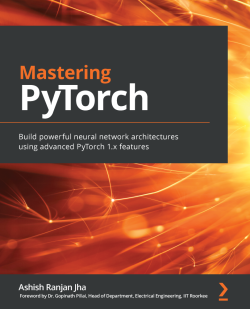# Mastering PyTorch

By Ashish Ranjan Jha
• \$33.99 eBook
• \$46.99 Print + eBook
What do you get with a Packt Subscription?

• Instant access to this title and 7,500+ eBooks & Videos
• Constantly updated with 100+ new titles each month
• Breadth and depth in over 1,000+ technologies

Deep learning is driving the AI revolution, and PyTorch is making it easier than ever before for anyone to build deep learning applications. This PyTorch book will help you uncover expert techniques to get the most out of your data and build complex neural network models.

The book starts with a quick overview of PyTorch and explores using convolutional neural network (CNN) architectures for image classification. You'll then work with recurrent neural network (RNN) architectures and transformers for sentiment analysis. As you advance, you'll apply deep learning across different domains, such as music, text, and image generation using generative models and explore the world of generative adversarial networks (GANs). You'll not only build and train your own deep reinforcement learning models in PyTorch but also deploy PyTorch models to production using expert tips and techniques. Finally, you'll get to grips with training large models efficiently in a distributed manner, searching neural architectures effectively with AutoML, and rapidly prototyping models using PyTorch and fast.ai.

By the end of this PyTorch book, you'll be able to perform complex deep learning tasks using PyTorch to build smart artificial intelligence models.

Publication date:
February 2021
Publisher
Packt
Pages
450
ISBN
9781789614381

# Chapter 1: Overview of Deep Learning using PyTorch

Deep learning is a class of machine learning methods that has revolutionized the way computers/machines are used to perform cognitive tasks in real life. Based on the mathematical concept of deep neural networks, deep learning uses large amounts of data to learn non-trivial relationships between inputs and outputs in the form of complex nonlinear functions. Some of the inputs and outputs, as demonstrated in Figure 1.1, could be the following:

• Input: An image of a text; output: Text
• Input: Text; output: A natural voice speaking the text
• Input: A natural voice speaking the text; output: Transcribed text

And so on. Here is a figure to support the preceding explanation: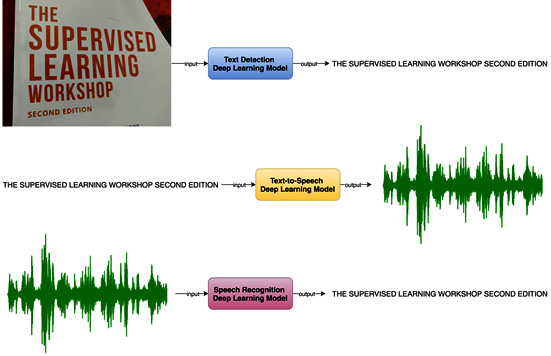Figure 1.1 – Deep learning model examples

Deep neural networks involve a lot of mathematical computations, linear algebraic equations, complex nonlinear functions, and various optimization algorithms. In order to build and train a deep neural network from scratch using a programming language such as Python, it would require us to write all the necessary equations, functions, and optimization schedules. Furthermore, the code would need to be written such that large amounts of data can be loaded efficiently, and training can be performed in a reasonable amount of time. This amounts to implementing several lower-level details each time we build a deep learning application.

Deep learning libraries such as Theano and TensorFlow, among various others, have been developed over the years to abstract these details out. PyTorch is one such Python-based deep learning library that can be used to build deep learning models.

TensorFlow was introduced as an open source deep learning Python (and C++) library by Google in late 2015, which revolutionized the field of applied deep learning. Facebook, in 2016, responded with its own open source deep learning library and called it Torch. Torch was initially used with a scripting language called Lua, and soon enough, the Python equivalent emerged called PyTorch. Around the same time, Microsoft released its own library – CNTK. Amidst the hot competition, PyTorch has been growing fast to become one of the most used deep learning libraries.

This book is meant to be a hands-on resource on some of the most advanced deep learning problems, how they are solved using complex deep learning architectures, and how PyTorch can be effectively used to build, train, and evaluate these complex models. While the book keeps PyTorch at the center, it also includes comprehensive coverage of some of the most recent and advanced deep learning models. The book is intended for data scientists, machine learning engineers, or researchers who have a working knowledge of Python and who, preferably, have used PyTorch before.

Due to the hands-on nature of this book, it is highly recommended to try the examples in each chapter by yourself on your computer to become proficient in writing PyTorch code. We begin with this introductory chapter and subsequently explore various deep learning problems and model architectures that will expose the various functionalities PyTorch has to offer.

This chapter will review some of the concepts behind deep learning and will provide a brief overview of the PyTorch library. We conclude this chapter with a hands-on exercise where we train a deep learning model using PyTorch.

The following topics will be covered in this chapter:

• A refresher on deep learning
• Exploring the PyTorch library
• Training a neural network using PyTorch

# Technical requirements

We will be using Jupyter notebooks for all of our exercises. And the following is the list of Python libraries that shall be installed for this chapter using `pip`. For example, run `pip install torch==1.4.0` on the command line:

```jupyter==1.0.0
torch==1.4.0
torchvision==0.5.0
matplotlib==3.1.2```

All code files relevant to this chapter are available at https://github.com/PacktPublishing/Mastering-PyTorch/tree/master/Chapter01.

# A refresher on deep learning

Neural networks are a sub-type of machine learning methods that are inspired by the structure and function of the human brain. In neural networks, each computational unit, analogically called a neuron, is connected to other neurons in a layered fashion. When the number of such layers is more than two, the neural network thus formed is called a deep neural network. Such models are generally called deep learning models.

Deep learning models have proven superior to other classical machine learning models because of their ability to learn highly complex relationships between input data and the output (ground truth). In recent times, deep learning has gained a lot of attention and rightly so, primarily because of the following two reasons:

• The availability of powerful computing machines, especially in the cloud
• The availability of huge amounts of data

Owing to Moore's law, which states that the processing power of computers will double every 2 years, we are now living in a time when deep learning models with several hundreds of layers can be trained within a realistic and reasonably short amount of time. At the same time, with the exponential increase in the use of digital devices everywhere, our digital footprint has exploded, resulting in gigantic amounts of data being generated across the world every moment.

Hence, it has been possible to train deep learning models for some of the most difficult cognitive tasks that were either intractable earlier or had sub-optimal solutions through other machine learning techniques.

Deep learning, or neural networks in general, has another advantage over the classical machine learning models. Usually, in a classical machine learning-based approach, feature engineering plays a crucial role in the overall performance of a trained model. However, a deep learning model does away with the need to manually craft features. With large amounts of data, deep learning models can perform very well without requiring hand-engineered features and can outperform the traditional machine learning models. The following graph indicates how deep learning models can leverage large amounts of data better than the classical machine models: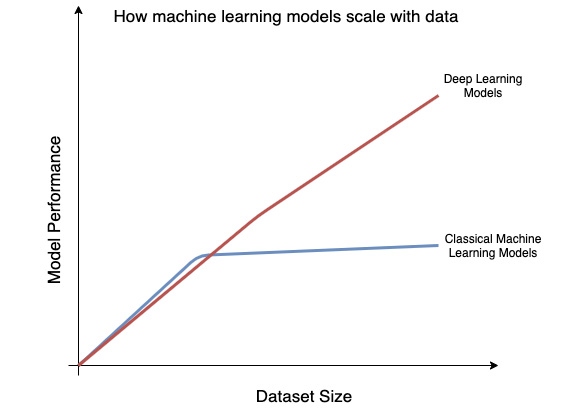Figure 1.2 – Model performance versus dataset size

As can be seen in the graph, deep learning performance isn't necessarily distinguished up to a certain dataset size. However, as the data size starts to further increase, deep neural networks begin outperforming the non-deep learning models.

A deep learning model can be built based on various types of neural network architectures that have been developed over the years. A prime distinguishing factor between the different architectures is the type and combination of layers that are used in the neural network. Some of the well-known layers are the following:

• Fully-connected or linear: In a fully connected layer, as shown in the following diagram, all neurons preceding this layer are connected to all neurons succeeding this layer: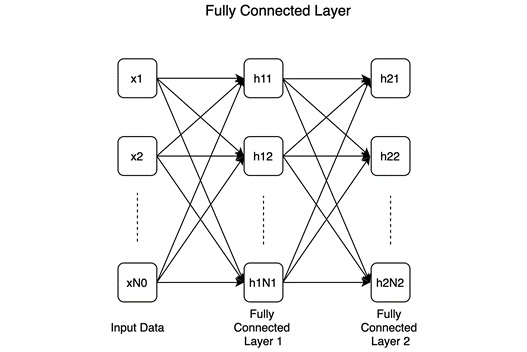Figure 1.3 – Fully connected layer

This example shows two consecutive fully connected layers with N1 and N2 number of neurons, respectively. Fully connected layers are a fundamental unit of many – in fact, most – deep learning classifiers.

• Convolutional: The following diagram shows a convolutional layer, where a convolutional kernel (or filter) is convolved over the input: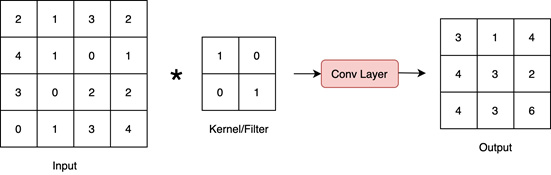Figure 1.4 – Convolutional layer

Convolutional layers are a fundamental unit of convolutional neural networks (CNNs), which are the most effective models for solving computer vision problems.

• Recurrent: The following diagram shows a recurrent layer. While it looks similar to a fully connected layer, the key difference is the recurrent connection (marked with bold curved arrows):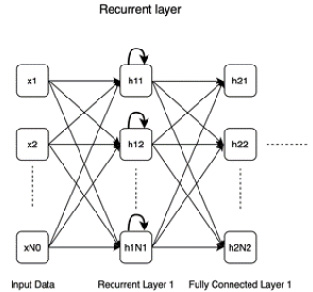Figure 1.5 – Recurrent layer

Recurrent layers have an advantage over fully connected layers in that they exhibit memorizing capabilities, which comes in handy working with sequential data where one needs to remember past inputs along with the present inputs.

• DeConv (the reverse of a convolutional layer): Quite the opposite of a convolutional layer, a deconvolutional layer works as shown in the following diagram: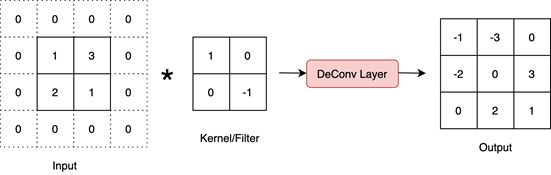Figure 1.6 – Deconvolutional layer

This layer expands the input data spatially and hence is crucial in models that aim to generate or reconstruct images, for example.

• Pooling: The following diagram shows the max-pooling layer, which is perhaps the most widely used kind of pooling layer: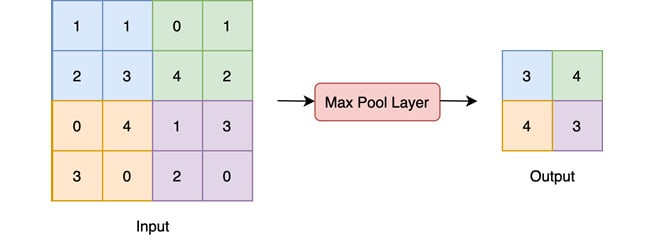Figure 1.7 – Pooling layer

This is a max-pooling layer that pools the highest number each from 2x2 sized subsections of the input. Other forms of pooling are min-pooling and mean-pooling.

• Dropout: The following diagram shows how dropout layers work. Essentially, in a dropout layer, some neurons are temporarily switched off (marked with X in the diagram), that is, they are disconnected from the network: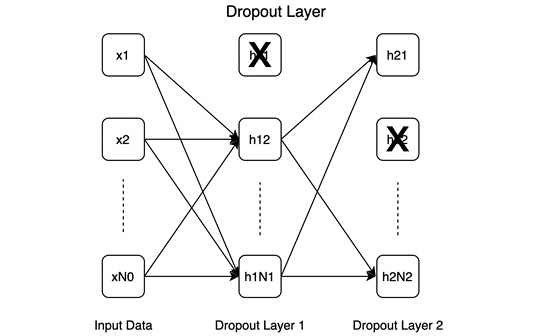Figure 1.8 – Dropout layer

Dropout helps in model regularization as it forces the model to function well in sporadic absences of certain neurons, which forces the model to learn generalizable patterns instead of memorizing the entire training dataset.

A number of well-known architectures based on the previously mentioned layers are shown in the following diagram:.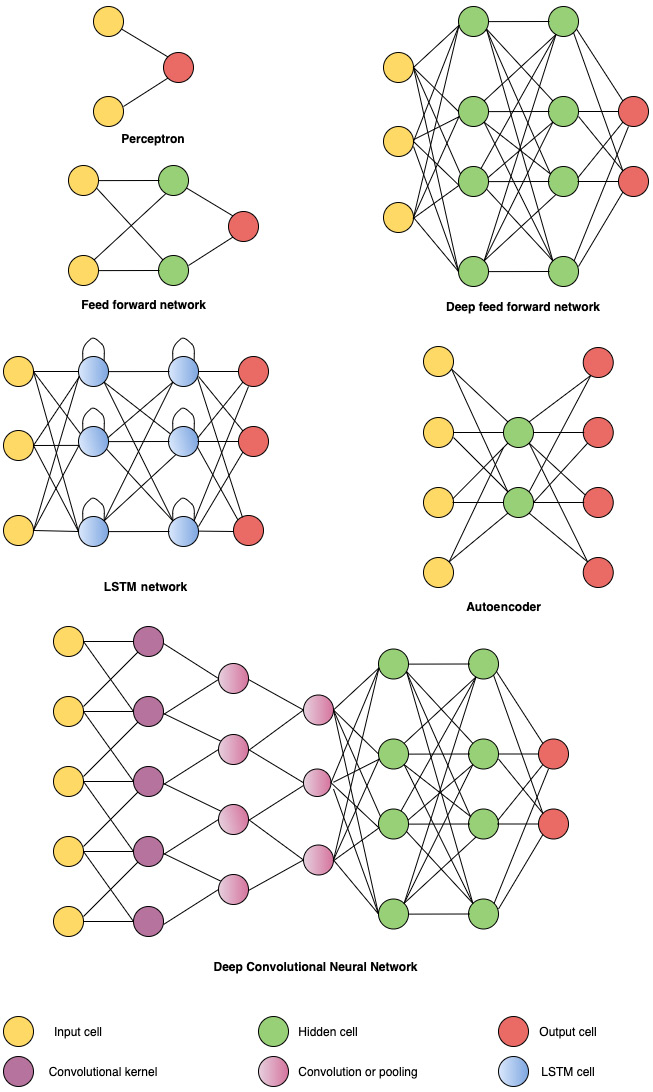Figure 1.9 – Different neural network architectures

A more exhaustive set of neural network architectures can be found here: https://www.asimovinstitute.org/neural-network-zoo/.

Besides the types of layers and how they are connected in a network, other factors such as activation functions and the optimization schedule also define the model.

## Activation functions

Activation functions are crucial to neural networks as they add the non-linearity without which, no matter how many layers we add, the entire neural network would be reduced to a simple linear model. The different types of activation functions listed here are basically different nonlinear mathematical functions.

Some of the popular activation functions are as follows:

• Sigmoid: A sigmoid (or logistic) function is expressed as follows: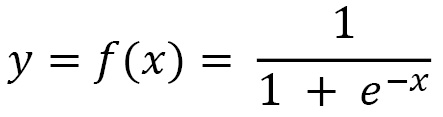The function is shown in graph form as follows: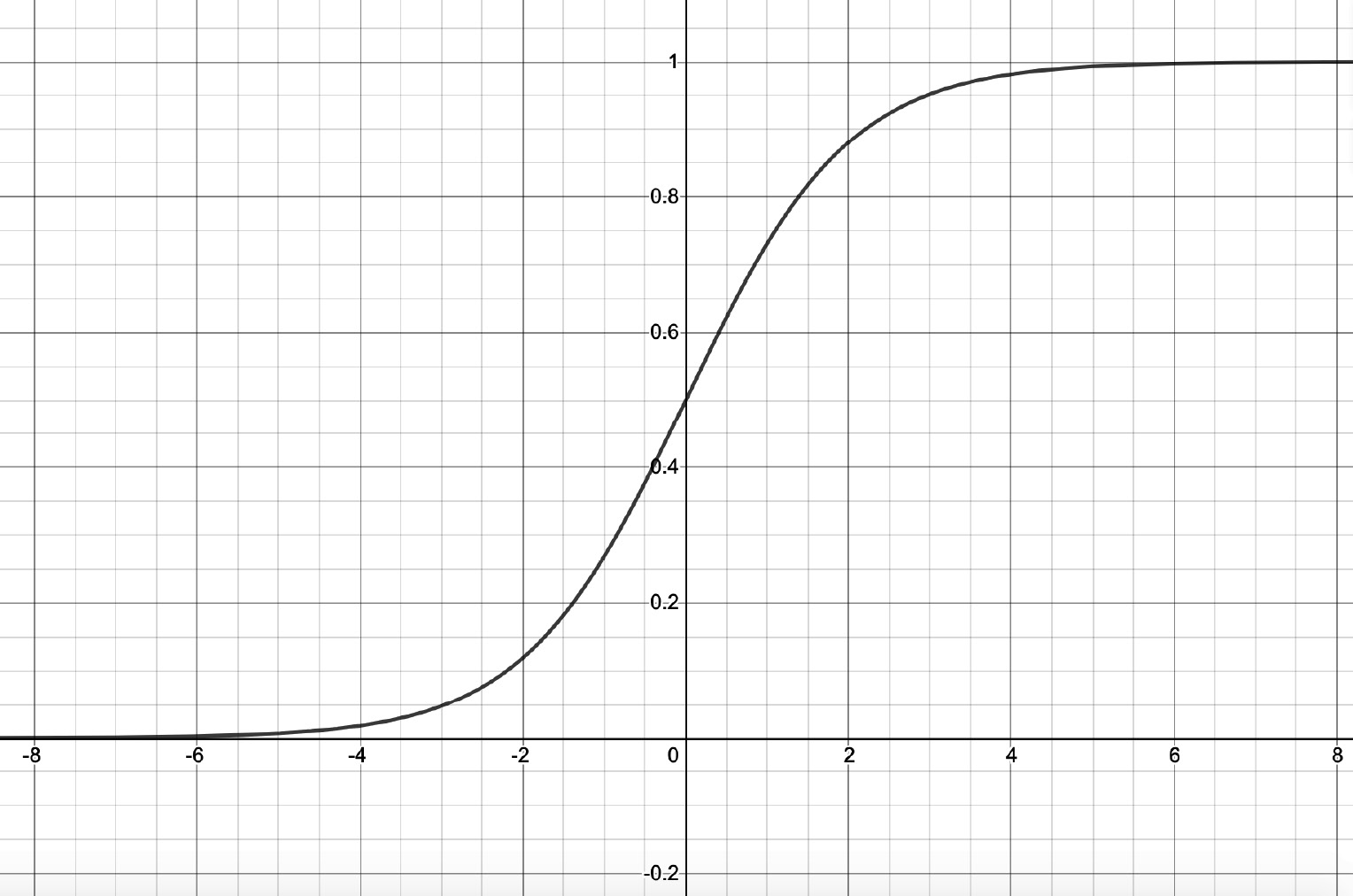Figure 1.10 – Sigmoid function

As can be seen from the graph, the sigmoid function takes in a numerical value x as input and outputs a value y in the range (0, 1).

• TanH: TanH is expressed as follows: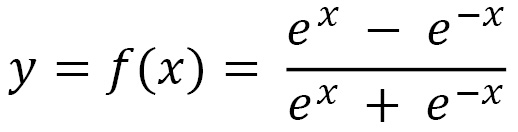The function is shown in graph form as follows: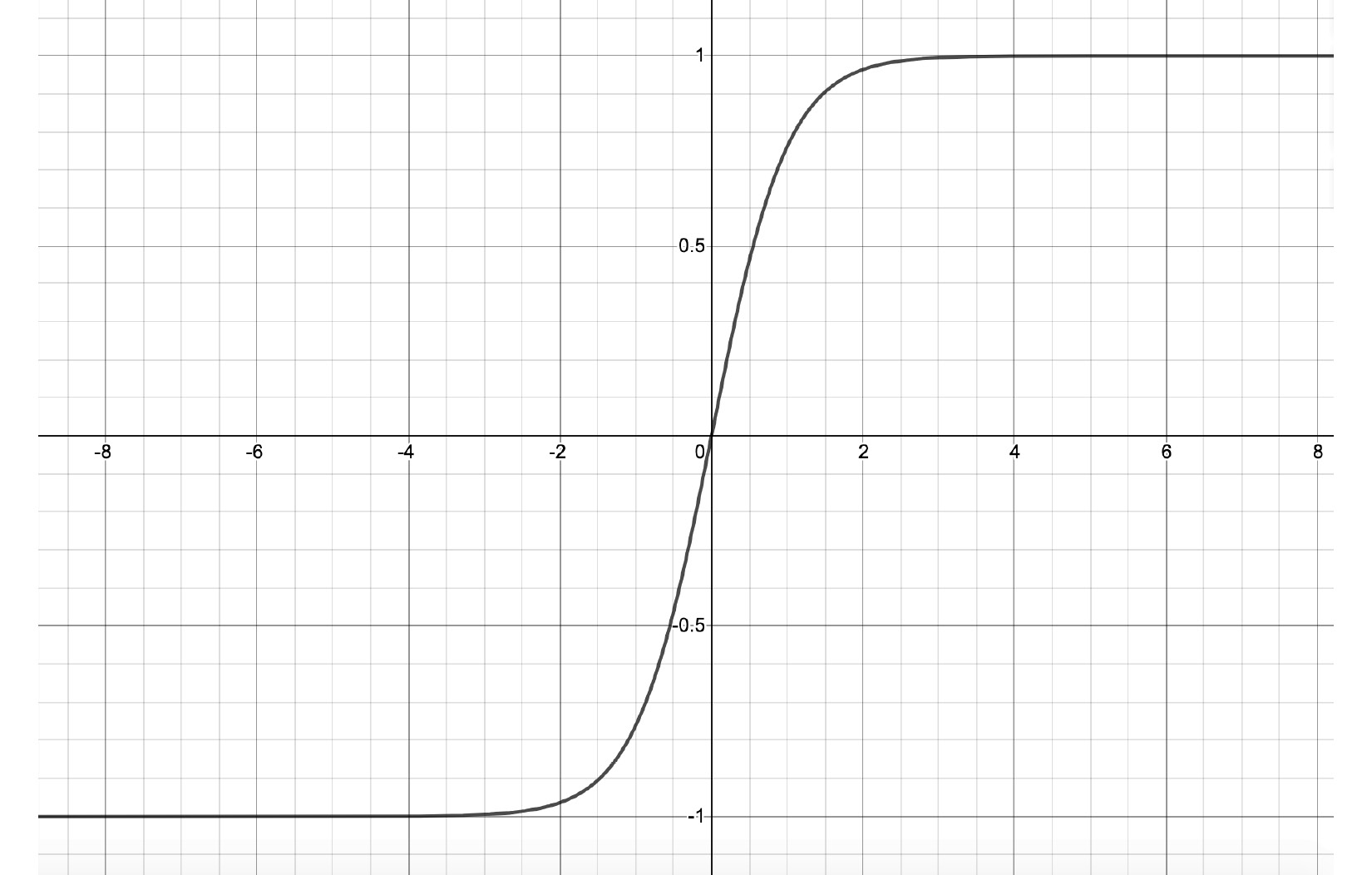Figure 1.11 – TanH function

Contrary to sigmoid, the output y varies from -1 to 1 in the case of the TanH activation function. Hence, this activation is useful in cases where we need both positive as well as negative outputs.

• Rectified linear units (ReLUs): ReLUs are more recent than the previous two and are simply expressed as follows: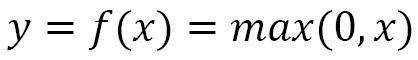The function is shown in graph form as follows: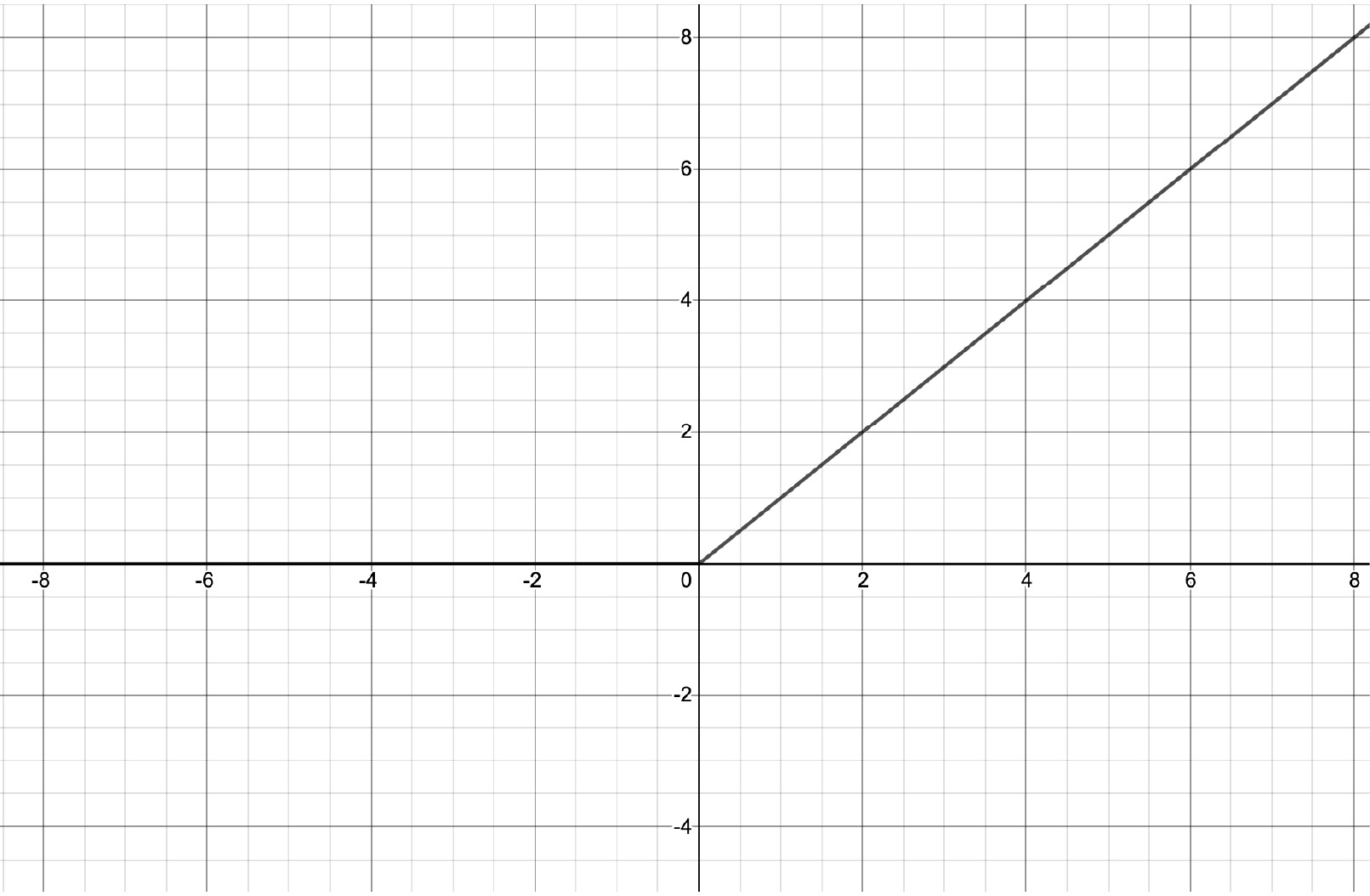Figure 1.12 – ReLU function

A distinct feature of ReLU in comparison with the sigmoid and TanH activation functions is that the output keeps growing with the input whenever the input is greater than 0. This prevents the gradient of this function from diminishing to 0 as in the case of the previous two activation functions. Although, whenever the input is negative, both the output and the gradient will be 0.

• Leaky ReLU: ReLUs entirely suppress any incoming negative input by outputting 0. We may, however, want to also process negative inputs for some cases. Leaky ReLUs offer the option of processing negative inputs by outputting a fraction k of the incoming negative input. This fraction k is a parameter of this activation function, which can be mathematically expressed as follows: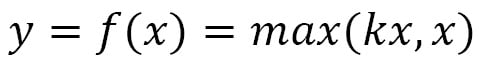The following graph shows the input-output relationship for leaky ReLU: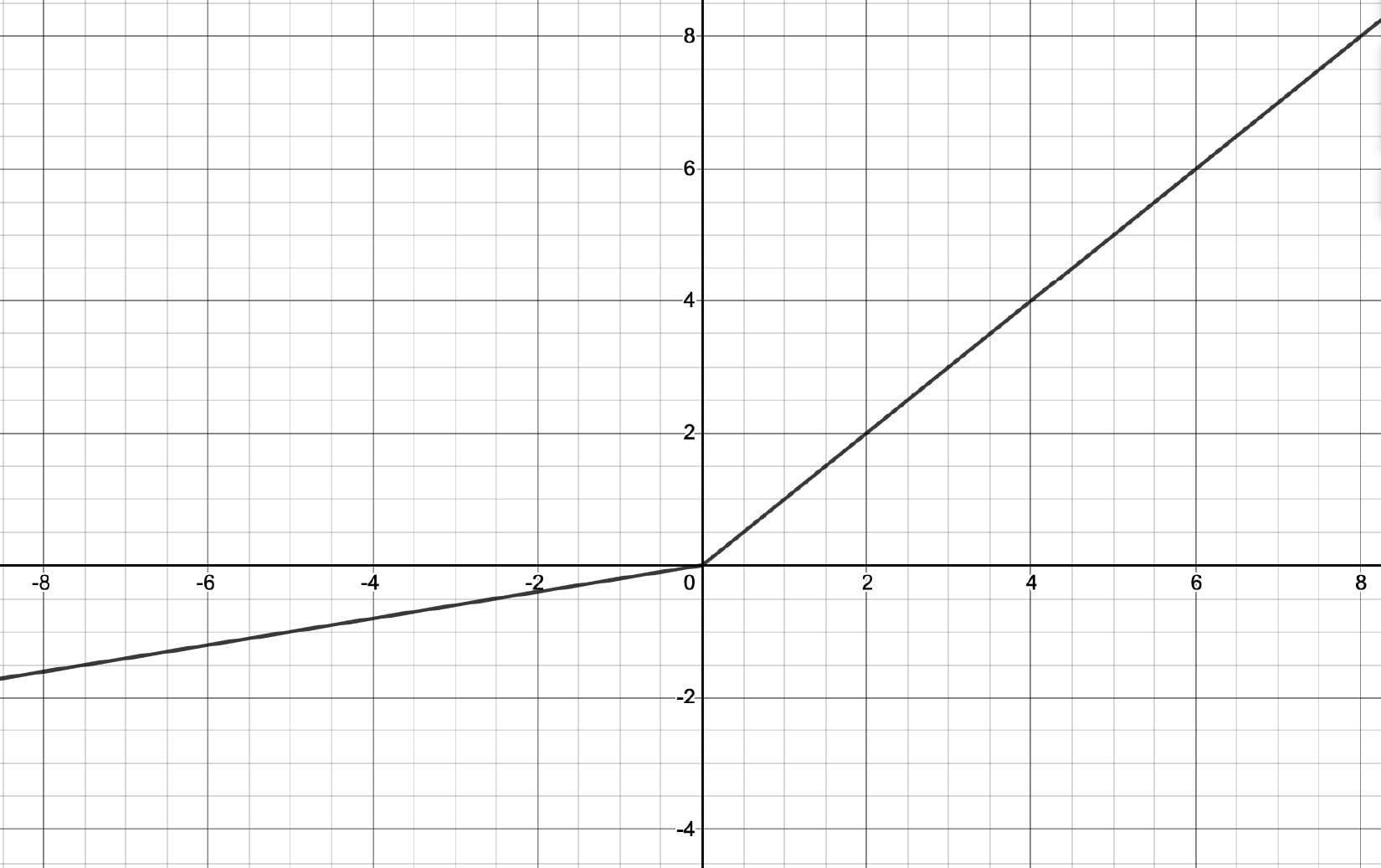Figure 1.13 – Leaky ReLU function

Activation functions are an actively evolving area of research within deep learning. It will not be possible to list all of the activation functions here but I encourage you to check out the recent developments in this domain. Many activation functions are simply nuanced modifications of the ones mentioned in this section.

## Optimization schedule

So far, we have spoken of how a neural network structure is built. In order to train a neural network, we need to adopt an optimization schedule. Like any other parameter-based machine learning model, a deep learning model is trained by tuning its parameters. The parameters are tuned through the process of backpropagation, wherein the final or output layer of the neural network yields a loss. This loss is calculated with the help of a loss function that takes in the neural network's final layer's outputs and the corresponding ground truth target values. This loss is then backpropagated to the previous layers using gradient descent and the chain rule of differentiation.

The parameters or weights at each layer are accordingly modified in order to minimize the loss. The extent of modification is determined by a coefficient, which varies from 0 to 1, also known as the learning rate. This whole procedure of updating the weights of a neural network, which we call the optimization schedule, has a significant impact on how well a model is trained. Therefore, a lot of research has been done in this area and is still ongoing. The following are a few popular optimization schedules:

• Stochastic Gradient Descent (SGD): It updates the model parameters in the following fashion: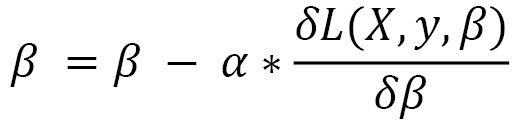β is the parameter of the model and X and y are the input training data and the corresponding labels respectively. L is the loss function and α is the learning rate. SGD performs this update for every training example pair (X, y). A variant of this –mini-batch gradient descent – performs updates for every k examples, where k is the batch size. Gradients are calculated altogether for the whole mini-batch. Another variant, batch gradient descent, performs parameter updates by calculating the gradient across the entire dataset.

• Adagrad: In the previous optimization schedule, we used a single learning rate for all the parameters of the model. However, different parameters might need to be updated at different paces, especially in cases of sparse data, where some parameters are more actively involved in feature extraction than others. Adagrad introduces the idea of per-parameter updates, as shown here: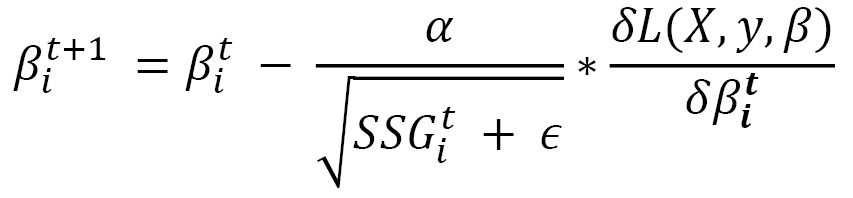Here, we use the subscript i to denote the ith parameter and the superscript t is used to denote the time step t of the gradient descent iterations. SSGit is the sum of squared gradients for the ith parameter starting from time step 0 to time step t. є is used to denote a small value added to SSG to avoid division by zero. Dividing the global learning rate α by the square root of SSG ensures that the learning rate for frequently changing parameters lowers faster than the learning rate for rarely updated parameters.

• Adadelta: In Adagrad, the denominator of the learning rate is a term that keeps on rising in value due to added squared terms in every time step. This causes the learning rates to decay to vanishingly small values. To tackle this problem, Adadelta introduces the idea of computing the sum of squared gradients only up to previous time steps. In fact, we can express it as a running decaying average of the past gradients: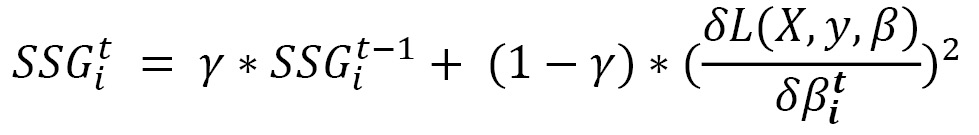γ here is the decaying factor we wish to choose for the previous sum of squared gradients. With this formulation, we ensure that the sum of squared gradients does not accumulate to a large value, thanks to the decaying average. Once SSGit is defined, we can use the Adagrad equation to define the update step for Adadelta.

However, if we look closely at the Adagrad equation, the root mean squared gradient is not a dimensionless quantity and hence should ideally not be used as a coefficient for the learning rate. To resolve this, we define another running average, this time for the squared parameter updates. Let's first define the parameter update:And then, similar to the running decaying average of the past gradients equation (the first equation under Adadelta), we can define the square sum of parameter updates as follows: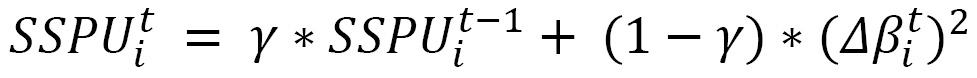Here, SSPU is the sum of squared parameter updates. Once we have this, we can adjust for the dimensionality problem in the Adagrad equation with the final Adadelta equation: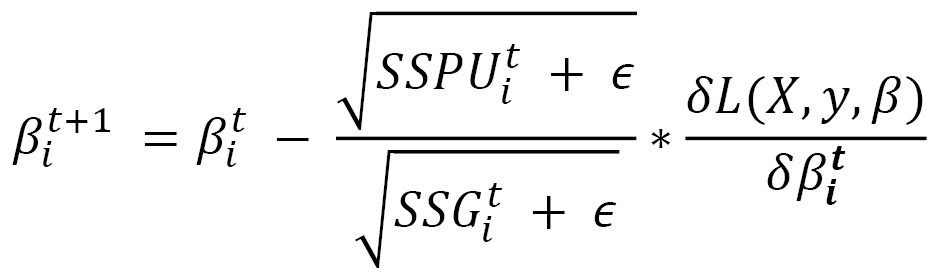Noticeably, the final Adadelta equation doesn't require any learning rate. One can still however provide a learning rate as a multiplier. Hence, the only mandatory hyperparameter for this optimization schedule is the decaying factors..

• RMSprop: We have implicitly discussed the internal workings of RMSprop while discussing Adadelta as both are pretty similar. The only difference is that RMSProp does not adjust for the dimensionality problem and hence the update equation stays the same as the equation presented in the Adagrad section, wherein the SSGit is obtained from the first equation in the Adadelta section. This essentially means that we do need to specify both a base learning rate as well as a decaying factor in the case of RMSProp.
• Adaptive Moment Estimation (Adam): This is another optimization schedule that calculates customized learning rates for each parameter. Just like Adadelta and RMSprop, Adam also uses the decaying average of the previous squared gradients as demonstrated in the first equation in the Adadelta section. However, it also uses the decaying average of previous gradient values: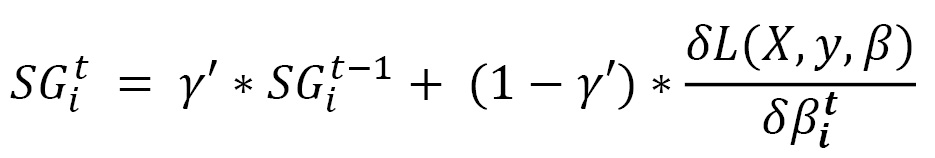SG and SSG are mathematically equivalent to estimating the first and second moments of the gradient respectively, hence the name of this method – adaptive moment estimation. Usually, γ and γ' are close to 1 and in that case, the initial values for both SG and SSG might be pushed towards zero. To counteract that, these two quantities are reformulated with the help of bias correction: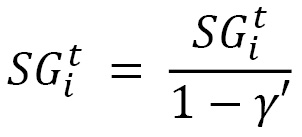and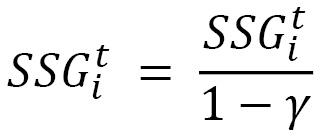Once they are defined, the parameter update is expressed as follows: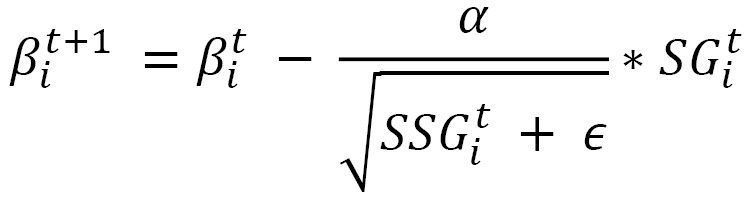Basically, the gradient on the extreme right-hand side of the equation is replaced by the decaying average of the gradient. Noticeably, Adam optimization involves three hyperparameters – the base learning rate, and the two decaying rates for the gradients and squared gradients. Adam is one of the most successful, if not the most successful, optimization schedule in recent times for training complex deep learning models.

So, which optimizer shall we use? It depends. If we are dealing with sparse data, then the adaptive optimizers (numbers 2 to 5) will be advantageous because of the per-parameter learning rate updates. As mentioned earlier, with sparse data, different parameters might be worked at different paces and hence a customized per-parameter learning rate mechanism can greatly help the model in reaching optimal solutions. SGD might also find a decent solution but will take much longer in terms of training time. Among the adaptive ones, Adagrad has the disadvantage of vanishing learning rates due to a monotonically increasing learning rate denominator.

RMSProp, Adadelta, and Adam are quite close in terms of their performance on various deep learning tasks. RMSprop is largely similar to Adadelta, except for the use of the base learning rate in RMSprop versus the use of the decaying average of previous parameter updates in Adadelta. Adam is slightly different in that it also includes the first-moment calculation of gradients and accounts for bias correction. Overall, Adam could be the optimizer to go with, all else being equal. We will use some of these optimization schedules in the exercises in this book. Feel free to switch them with another one to observe changes in the following:

• Model training time and trajectory (convergence)
• Final model performance

In the coming chapters, we will use many of these architectures, layers, activation functions, and optimization schedules in solving different kinds of machine learning problems with the help of PyTorch. In the example included in this chapter, we will create a convolutional neural network that contains convolutional, linear, max-pooling, and dropout layers. Log-Softmax is used for the final layer and ReLU is used as the activation function for all the other layers. And the model is trained using an Adadelta optimizer with a fixed learning rate of `0.5`.

# Exploring the PyTorch library

PyTorch is a machine learning library for Python based on the Torch library. PyTorch is extensively used as a deep learning tool both for research as well as building industrial applications. It is primarily developed by Facebook's machine learning research labs. PyTorch is competition for the other well-known deep learning library – TensorFlow, which is developed by Google. The initial difference between these two was that PyTorch was based on eager execution whereas TensorFlow was built on graph-based deferred execution. Although, TensorFlow now also provides an eager execution mode.

Eager execution is basically an imperative programming mode where mathematical operations are computed immediately. A deferred execution mode would have all the operations stored in a computational graph without immediate calculations and then the entire graph would be evaluated later. Eager execution is considered advantageous for reasons such as intuitive flow, easy debugging, and less scaffolding code.

PyTorch is more than just a deep learning library. With its NumPy-like syntax/interface, it provides tensor computation capabilities with strong acceleration using GPUs. But what is a tensor? Tensors are computational units, very similar to NumPy arrays, except that they can also be used on GPUs to accelerate computing.

With accelerated computing and the facility to create dynamic computational graphs, PyTorch provides a complete deep learning framework. Besides all that, it is truly Pythonic in nature, which enables PyTorch users to exploit all the features Python provides, including the extensive Python data science ecosystem.

In this section, we will take a look at some of the useful PyTorch modules that extend various functionalities helpful in loading data, building models, and specifying the optimization schedule during the training of a model. We will also expand on what a tensor is and how it is implemented with all of its attributes in PyTorch.

## PyTorch modules

The PyTorch library, besides offering the computational functions as NumPy does, also offers a set of modules that enable developers to quickly design, train, and test deep learning models. The following are some of the most useful modules.

### torch.nn

When building a neural network architecture, the fundamental aspects that the network is built on are the number of layers, the number of neurons in each layer, and which of those are learnable, and so on. The PyTorch `nn` module enables users to quickly instantiate neural network architectures by defining some of these high-level aspects as opposed to having to specify all the details manually. The following is a one-layer neural network initialization without using the `nn` module:

```import math
# we assume a 256-dimensional input and a 4-dimensional output for this 1-layer neural network
# hence, we initialize a 256x4 dimensional matrix filled with random values
weights = torch.randn(256, 4) / math.sqrt(256)
# we then ensure that the parameters of this neural network ar trainable, that is, the numbers in the 256x4 matrix can be tuned with the help of backpropagation of gradients
# finally we also add the bias weights for the 4-dimensional output, and make these trainable too
bias = torch.zeros(4, requires_grad=True)```

We can instead use `nn.Linear(256, 4)` to represent the same thing.

Within the `torch.nn` module, there is a submodule called `torch.nn.functional`. This submodule consists of all the functions within the `torch.nn` module whereas all the other submodules are classes. These functions are loss functions, activating functions, and also neural functions that can be used to create neural networks in a functional manner (that is, when each subsequent layer is expressed as a function of the previous layer) such as pooling, convolutional, and linear functions. An example of a loss function using the `torch.nn.functional` module could be the following:

```import torch.nn.functional as F
loss_func = F.cross_entropy
loss = loss_func(model(X), y)```

Here, `X` is the input, `y` is the target output, and model is the neural network model.

### torch.optim

As we train a neural network, we back-propagate errors to tune the weights or parameters of the network – the process that we call optimization. The `optim` module includes all the tools and functionalities related to running various types of optimization schedules while training a deep learning model. Let's say we define an optimizer during a training session using the `torch.optim` modules, as shown in the following snippet:

`opt = optim.SGD(model.parameters(), lr=lr)`

Then, we don't need to manually write the optimization step as shown here:

```with torch.no_grad():
# applying the parameter updates using stochastic gradient descent
for param in model.parameters(): param -= param.grad * lr

We can simply write this instead:

```opt.step()

Next, we will look at the `utis.data` module.

### torch.utils.data

Under the `utis.data` module, torch provides its own dataset and `DatasetLoader` classes, which are extremely handy due to their abstract and flexible implementations. Basically, these classes provide intuitive and useful ways of iterating and performing other such operations on tensors. Using these, we can ensure high performance due to optimized tensor computations and also have fail-safe data I/O. For example, let's say we use `torch.utils.data.DataLoader` as follows:

```from torch.utils.data import (TensorDataset, DataLoader)
train_dataset = TensorDataset(x_train, y_train)

Then, we don't need to iterate through batches of data manually, like this:

```for i in range((n-1)//bs + 1):
x_batch = x_train[start_i:end_i]
y_batch = y_train[start_i:end_i]
pred = model(x_batch)```

We can simply write this instead:

```for x_batch,y_batch in train_dataloader:
pred = model(x_batch)```

Let's now look at tensor modules.

## Tensor modules

As mentioned earlier, tensors are conceptually similar to NumPy arrays. A tensor is an n-dimensional array on which we can operate mathematical functions, accelerate computations via GPUs, and tensors can also be used to keep track of a computational graph and gradients, which prove vital for deep learning. To run a tensor on a GPU, all we need is to cast the tensor into a certain data type.

Here is how we can instantiate a tensor in PyTorch:

`points = torch.tensor([1.0, 4.0, 2.0, 1.0, 3.0, 5.0]) `

To fetch the first entry, simply write the following:

`float(points)`

We can also check the shape of the tensor using this:

`points.shape`

In PyTorch, tensors are implemented as views over a one-dimensional array of numerical data stored in contiguous chunks of memory. These arrays are called storage instances. Every PyTorch tensor has a storage attribute that can be called to output the underlying storage instance for a tensor as shown in the following example:

```points = torch.tensor([[1.0, 4.0], [2.0, 1.0], [3.0, 5.0]])
points.storage()```

This should output the following: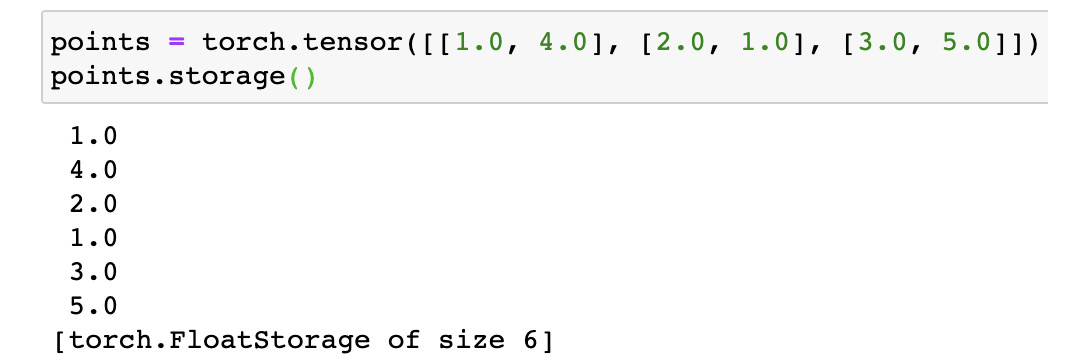Figure 1.14 – PyTorch tensor storage

When we say a tensor is a view on the storage instance, the tensor uses the following information to implement the view:

• Size
• Storage
• Offset
• Stride

Let's look into this with the help of our previous example:

`points = torch.tensor([[1.0, 4.0], [2.0, 1.0], [3.0, 5.0]])`

Let's investigate what these different pieces of information mean:

`points.size()`

This should output the following: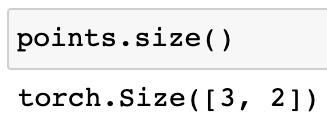Figure 1.15 – PyTorch tensor size

As we can see, `size` is similar to the `shape` attribute in NumPy, which tells us the number of elements across each dimension. The multiplication of these numbers equals the length of the underlying storage instance (6 in this case).

As we have already examined what the `storage` attribute means, let's look at `offset`:

`points.storage_offset()`

This should output the following: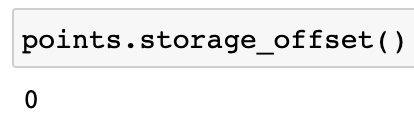Figure 1.16 – PyTorch tensor storage offset 1

The offset here represents the index of the first element of the tensor in the `storage` array. Because the output is `0`, it means that the first element of the tensor is the first element in the `storage` array.

Let's check this:

`points.storage_offset()`

This should output the following: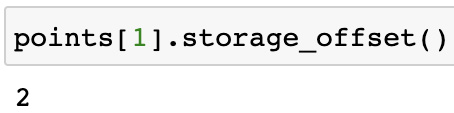Figure 1.17 – PyTorch tensor storage offset 2

Because `points` is `[2.0, 1.0]` and the `storage` array is `[1.0, 4.0, 2.0, 1.0, 3.0, 5.0]`, we can see that the first element of the tensor `[2.0, 1.0]`, that is, `. 2.0` is at index `2` of the `storage` array.

Finally, we'll look at the `stride` attribute:

`points.stride()`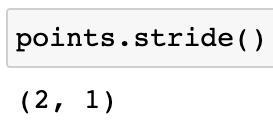Figure 1.18 – PyTorch tensor stride

As we can see, `stride` contains, for each dimension, the number of elements to be skipped in order to access the next element of the tensor. So, in this case, along the first dimension, in order to access the element after the first one, that is, `1.0` we need to skip 2 elements (that is, `1.0` and `4.0`) to access the next element, that is, `2.0`. Similarly, along the second dimension, we need to skip 1 element to access the element after `1.0`, that is, `4.0`. Thus, using all these attributes, tensors can be derived from a contiguous one-dimensional storage array.

The data contained within tensors is of numeric type. Specifically, PyTorch offers the following data types to be contained within tensors:

• `torch.float32` or `torch.float`—32-bit floating-point
• `torch.float64` or `torch.double`—64-bit, double-precision floating-point
• `torch.float16` or `torch.half`—16-bit, half-precision floating-point
• `torch.int8`—Signed 8-bit integers
• `torch.uint8`—Unsigned 8-bit integers
• `torch.int16` or `torch.short`—Signed 16-bit integers
• `torch.int32` or `torch.int`—Signed 32-bit integers
• `torch.int64` or `torch.long`—Signed 64-bit integers

An example of how we specify a certain data type to be used for a tensor is as follows:

`points = torch.tensor([[1.0, 2.0], [3.0, 4.0]], dtype=torch.float32)`

Besides the data type, tensors in PyTorch also need a device specification where they will be stored. A device can be specified as instantiation:

`points = torch.tensor([[1.0, 2.0], [3.0, 4.0]], dtype=torch.float32, device='cpu')`

Or we can also create a copy of a tensor in the desired device:

`points_2 = points.to(device='cuda')`

As seen in the two examples, we can either allocate a tensor to a CPU (using `device='cpu'`), which happens by default if we do not specify a device, or we can allocate the tensor to a GPU (using `device='cuda'`).

Note

PyTorch currently supports only GPUs that support CUDA.

When a tensor is placed on a GPU, the computations speed up and because the tensor APIs are largely uniform across CPU and GPU placed tensors in PyTorch, it is quite convenient to move the same tensor across devices, perform computations, and move it back.

If there are multiple devices of the same type, say more than one GPU, we can precisely locate the device we want to place the tensor in using the device index, such as the following:

`points_3 = points.to(device='cuda:0')`

You can read more about PyTorch-CUDA here: https://pytorch.org/docs/stable/notes/cuda.html. And you can read more generally about CUDA here: https://developer.nvidia.com/about-cuda.

Now that we have explored the PyTorch library and understood the PyTorch and Tensor modules, let's learn how to train a neural network using PyTorch.

# Training a neural network using PyTorch

For this exercise, we will be using the famous `MNIST` dataset (available at http://yann.lecun.com/exdb/mnist/), which is a sequence of images of handwritten postcode digits, zero through nine, with corresponding labels. The `MNIST` dataset consists of 60,000 training samples and 10,000 test samples, where each sample is a grayscale image with 28 x 28 pixels. PyTorch also provides the `MNIST` dataset under its `Dataset` module.

In this exercise, we will use PyTorch to train a deep learning multi-class classifier on this dataset and test how the trained model performs on the test samples:

1. For this exercise, we will need to import a few dependencies. Execute the following `import` statements:
```import torch
import torch.nn as nn
import torch.nn.functional as F
import torch.optim as optim
from torch.utils.data import DataLoader
from torchvision import datasets, transforms
import matplotlib.pyplot as plt```
2. Next, we define the model architecture as shown in the following diagram: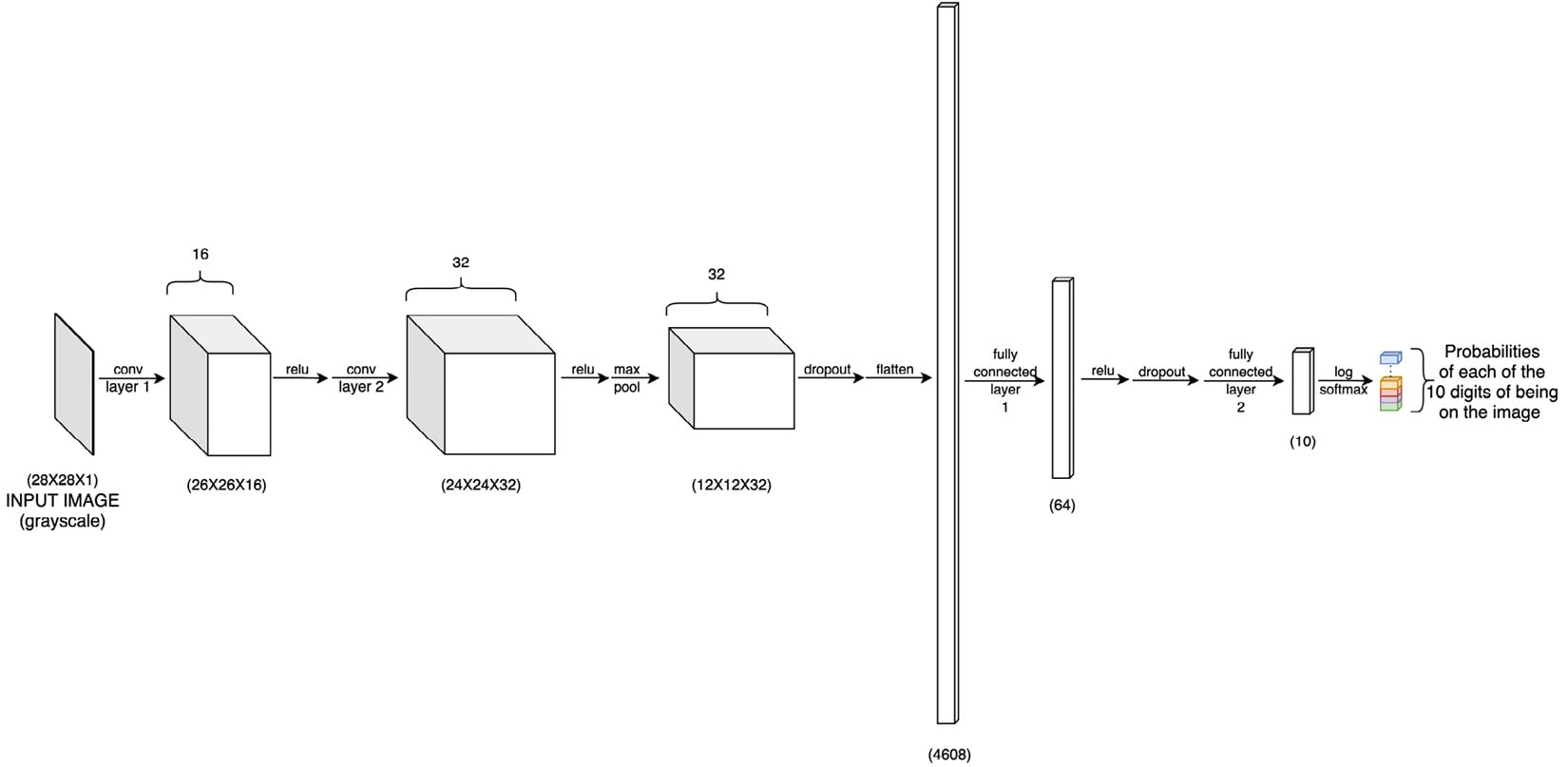Figure 1.19 – Neural network architecture

The model consists of convolutional layers, dropout layers, as well as linear/fully connected layers, all available through the `torch.nn` module:

```class ConvNet(nn.Module):
def __init__(self):
super(ConvNet, self).__init__()
self.cn1 = nn.Conv2d(1, 16, 3, 1)
self.cn2 = nn.Conv2d(16, 32, 3, 1)
self.dp1 = nn.Dropout2d(0.10)
self.dp2 = nn.Dropout2d(0.25)
self.fc1 = nn.Linear(4608, 64) # 4608 is basically 12 X 12 X 32
self.fc2 = nn.Linear(64, 10)
def forward(self, x):
x = self.cn1(x)
x = F.relu(x)
x = self.cn2(x)
x = F.relu(x)
x = F.max_pool2d(x, 2)
x = self.dp1(x)
x = torch.flatten(x, 1)
x = self.fc1(x)
x = F.relu(x)
x = self.dp2(x)
x = self.fc2(x)
op = F.log_softmax(x, dim=1)
return op```

The `__init__` function defines the core architecture of the model, that is, all the layers with the number of neurons at each layer. And the `forward` function, as the name suggests, does a forward pass in the network. Hence it includes all the activation functions at each layer as well as any pooling or dropout used after any layer. This function shall return the final layer output, which we call the prediction of the model, which has the same dimensions as the target output (the ground truth).

Notice that the first convolutional layer has a 1-channel input, a 16-channel output, a kernel size of 3, and a stride of 1. The 1-channel input is essentially for the grayscale images that will be fed to the model. We decided on a kernel size of 3x3 for various reasons. Firstly, kernel sizes are usually odd numbers so that the input image pixels are symmetrically distributed around a central pixel. 1x1 would be too small because then the kernel operating on a given pixel would not have any information about the neighboring pixels. 3 comes next, but why not go further to 5, 7, or, say, even 27?

Well, at the extreme high end, a 27x27 kernel convolving over a 28x28 image would give us very coarse-grained features. However, the most important visual features in the image are fairly local and hence it makes sense to use a small kernel that looks at a few neighboring pixels at a time, for visual patterns. 3x3 is one of the most common kernel sizes used in CNNs for solving computer vision problems.

Note that we have two consecutive convolutional layers, both with 3x3 kernels. This, in terms of spatial coverage, is equivalent to using one convolutional layer with a 5x5 kernel. However, using multiple layers with a smaller kernel size is almost always preferred because it results in deeper networks, hence more complex learned features as well as fewer parameters due to smaller kernels.

The number of channels in the output of a convolutional layer is usually higher than or equal to the input number of channels. Our first convolutional layer takes in one channel data and outputs 16 channels. This basically means that the layer is trying to detect 16 different kinds of information from the input image. Each of these channels is called a feature map and each of them has a dedicated kernel extracting features for them.

We escalate the number of channels from 16 to 32 in the second convolutional layer, in an attempt to extract more kinds of features from the image. This increment in the number of channels (or image depth) is common practice in CNNs. We will read more on this under width-based CNNs in Chapter 3, Deep CNN Architectures.

Finally, the stride of `1` makes sense, as our kernel size is just `3`. Keeping a larger stride value – say, 10 – would result in the kernel skipping many pixels in the image and we don't want to do that. If, however, our kernel size was 100, we might have considered 10 as a reasonable stride value. The larger the stride, the lower the number of convolution operations but the smaller the overall field of view for the kernel.

3. We then define the training routine, that is, the actual backpropagation step. As can be seen, the `torch.optim` module greatly helps in keeping this code succinct:
```def train(model, device, train_dataloader, optim, epoch):
model.train()
for b_i, (X, y) in enumerate(train_dataloader):
X, y = X.to(device), y.to(device)
pred_prob = model(X)
loss = F.nll_loss(pred_prob, y) # nll is the negative likelihood loss
loss.backward()
optim.step()
if b_i % 10 == 0:
print('epoch: {} [{}/{} ({:.0f}%)]\t training loss: {:.6f}'.format(
epoch, b_i * len(X), len(train_			dataloader.dataset),
100. * b_i / len(train_dataloader), loss.			item()))```

This iterates through the dataset in batches, makes a copy of the dataset on the given device, makes a forward pass with the retrieved data on the neural network model, computes the loss between the model prediction and the ground truth, uses the given optimizer to tune model weights, and prints training logs every 10 batches. The entire procedure done once qualifies as `1` epoch, that is, when the entire dataset has been read once.

4. Similar to the preceding training routine, we write a test routine that can be used to evaluate the model performance on the test set:
```def test(model, device, test_dataloader):
model.eval()
loss = 0
success = 0
for X, y in test_dataloader:
X, y = X.to(device), y.to(device)
pred_prob = model(X)
loss += F.nll_loss(pred_prob, y, reduction='sum').item()  # loss summed across the batch
pred = pred_prob.argmax(dim=1, 		 keepdim=True)  # us argmax to get the most 		 likely prediction
success += pred.eq(y.view_as(pred)).sum().item()
print('\nTest dataset: Overall Loss: {:.4f}, Overall Accuracy: {}/{} ({:.0f}%)\n'.format(
100. * success / len(test_dataloader.dataset)))```

Most of this function is similar to the preceding `train` function. The only difference is that the loss computed from the model predictions and the ground truth is not used to tune the model weights using an optimizer. Instead, the loss is used to compute the overall test error across the entire test batch.

5. Next, we come to another critical component of this exercise, which is loading the dataset. Thanks to PyTorch's `DataLoader` module, we can set up the dataset loading mechanism in a few lines of code:
```# The mean and standard deviation values are calculated as the mean of all pixel values of all images in the training dataset
transform=transforms.Compose([
transforms.ToTensor(),
transforms.Normalize((0.1302,), (0.3069,))])), # train_X.mean()/256. and train_X.std()/256.
batch_size=32, shuffle=True)
datasets.MNIST('../data', train=False,
transform=transforms.Compose([
transforms.ToTensor(),
transforms.Normalize((0.1302,), (0.3069,))
])),
batch_size=500, shuffle=False)```

As you can see, we set `batch_size` to `32`, which is a fairly common choice. Usually, there is a trade-off in deciding the batch size. A very small batch size can lead to slow training due to frequent gradient calculations and can lead to extremely noisy gradients. Very large batch sizes can, on the other hand, also slow down training due to a long waiting time to calculate gradients. It is mostly not worth waiting long before a single gradient update. It is rather advisable to make frequent, less precise gradients as it will eventually lead the model to a better set of learned parameters.

For both the training and test dataset, we specify the local storage location we want to save the dataset to, and the batch size, which determines the number of data instances that constitute one pass of a training and test run. We also specify that we want to randomly shuffle training data instances to ensure a uniform distribution of data samples across batches. Finally, we also normalize the dataset to a normal distribution with a specified mean and standard deviation.

6. We defined the training routine earlier. Now is the time to actually define which optimizer and device we will use to run the model training. And we will finally get the following:
```torch.manual_seed(0)
device = torch.device("cpu")
model = ConvNet()

We define the device for this exercise as `cpu`. We also set a seed to avoid unknown randomness and ensure repeatability. We will use AdaDelta as the optimizer for this exercise with a learning rate of `0.5`. While discussing optimization schedules earlier in the chapter, we mentioned that Adadelta could be a good choice if we are dealing with sparse data. And this is a case of sparse data, because not all pixels in the image are informative. Having said that, I encourage you to try out other optimizers such as Adam on this same problem to see how it affects the training process and model performance.

7. And then we start the actual process of training the model for k number of epochs, and we also keep testing the model at the end of each training epoch:
```for epoch in range(1, 3):
train(model, device, train_dataloader, optimizer, epoch)

For demonstration purposes, we will run the training for only two epochs. The output will be as follows: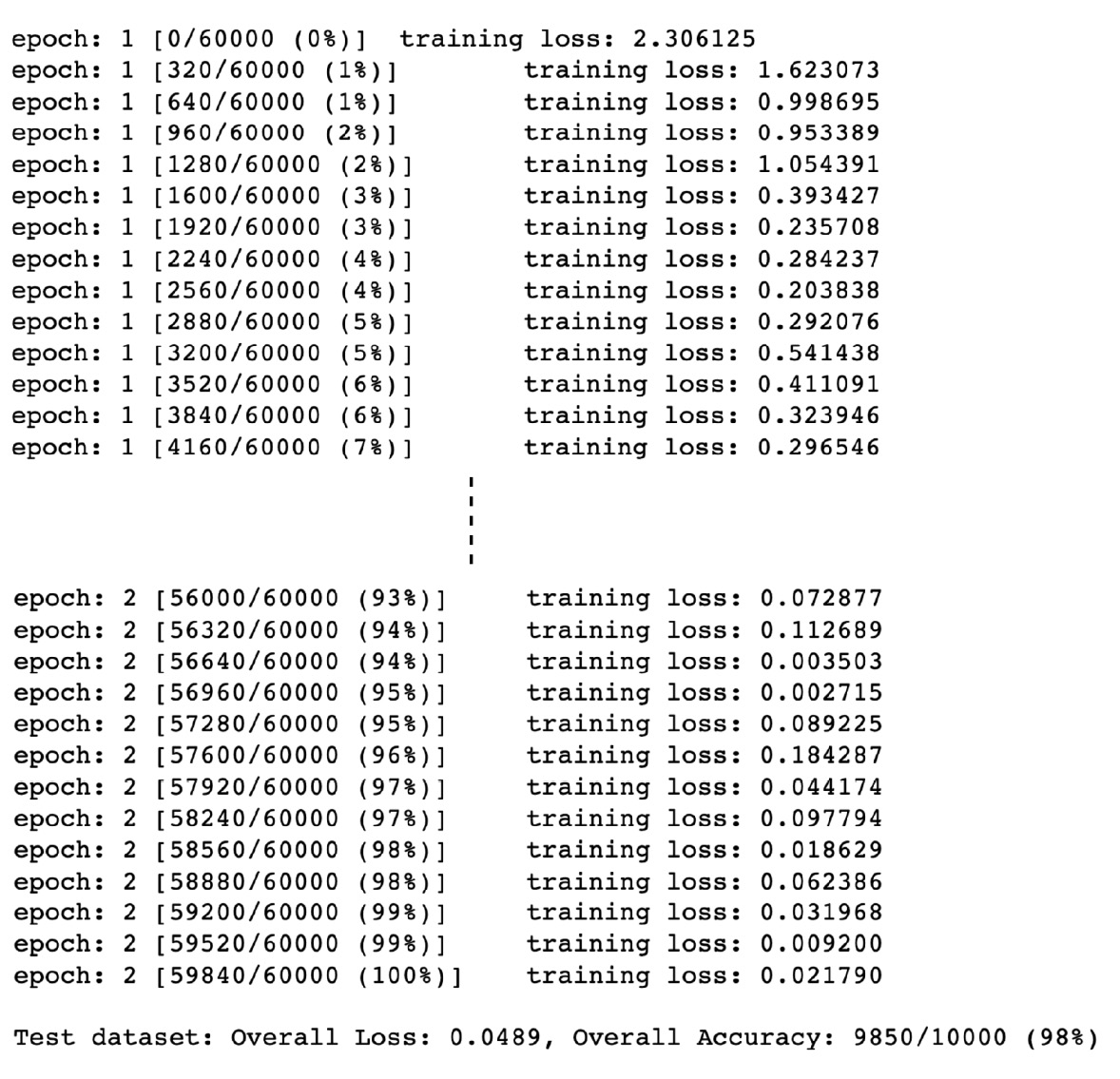Figure 1.20 – Training logs

8. Now that we have trained a model, with a reasonable test set performance, we can also manually check whether the model inference on a sample image is correct:
```test_samples = enumerate(test_dataloader)
b_i, (sample_data, sample_targets) = next(test_samples)
plt.imshow(sample_data, cmap='gray', interpolation='none')```

The output will be as follows: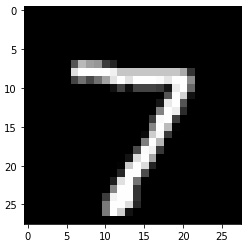Figure 1.21 – Sample handwritten image

And now we run the model inference for this image and compare it with the ground truth:

```     print(f"Model prediction is : {model(sample_data).data.max(1)}")
print(f"Ground truth is : {sample_targets}")```

Note that, for predictions, we first calculate the class with maximum probability using the `max` function on axis=1. The `max` function outputs two lists – a list of probabilities of classes for every sample in `sample_data` and a list of class labels for each sample. Hence, we choose the second list using index ``. We further select the first class label by using index `` to look at only the first sample under `sample_data`. The output will be as follows:Figure 1.22 – PyTorch model prediction

This appears to be the correct prediction. The forward pass of the neural network done using `model()` produces probabilities. Hence, we use the `max` function to output the class with the maximum probability.

Note

The code pattern for this exercise is derived from the official PyTorch examples repository, which can be found here: https://github.com/pytorch/examples/tree/master/mnist.

# Summary

In this chapter, we refreshed deep learning concepts such as layers, activation functions, and optimization schedules and how they contribute towards building varied deep learning architectures. We explored the PyTorch deep learning library, including some of the important modules, such as `torch.nn`, `torch.optim`, and `torch.data`, as well as tensor modules.

We then ran a hands-on exercise on training a deep learning model from scratch. We built a CNN for our exercise using PyTorch modules. We also wrote relevant PyTorch code to load the dataset, train and evaluate the model, and finally, make predictions from the trained model.

In the next chapter, we will explore a slightly more complex model architecture that involves multiple sub-models and use this type of hybrid model to tackle the real-world task of describing an image using natural text. Using PyTorch, we will implement such a system and generate captions for unseen images.

### About the Author

• ##### Ashish Ranjan Jha

Ashish Ranjan Jha received his bachelor’s degree in electrical engineering from IIT Roorkee (India), his master’s degree in computer science from EPFL (Switzerland), and an MBA degree from the Quantic School of Business (Washington). He received distinctions in all of his degrees. He has worked for a variety of tech companies, including Oracle and Sony, and tech start ups, such as Revolut, as a machine learning engineer.

Aside from his years of work experience, Ashish is a freelance ML consultant, an author, and a blogger (datashines). He has worked on products/projects ranging from using sensor data for predicting vehicle types to detecting fraud in insurance claims. In his spare time, Ashish works on open source ML projects and is active on StackOverflow and kaggle (arj7192).

Browse publications by this author